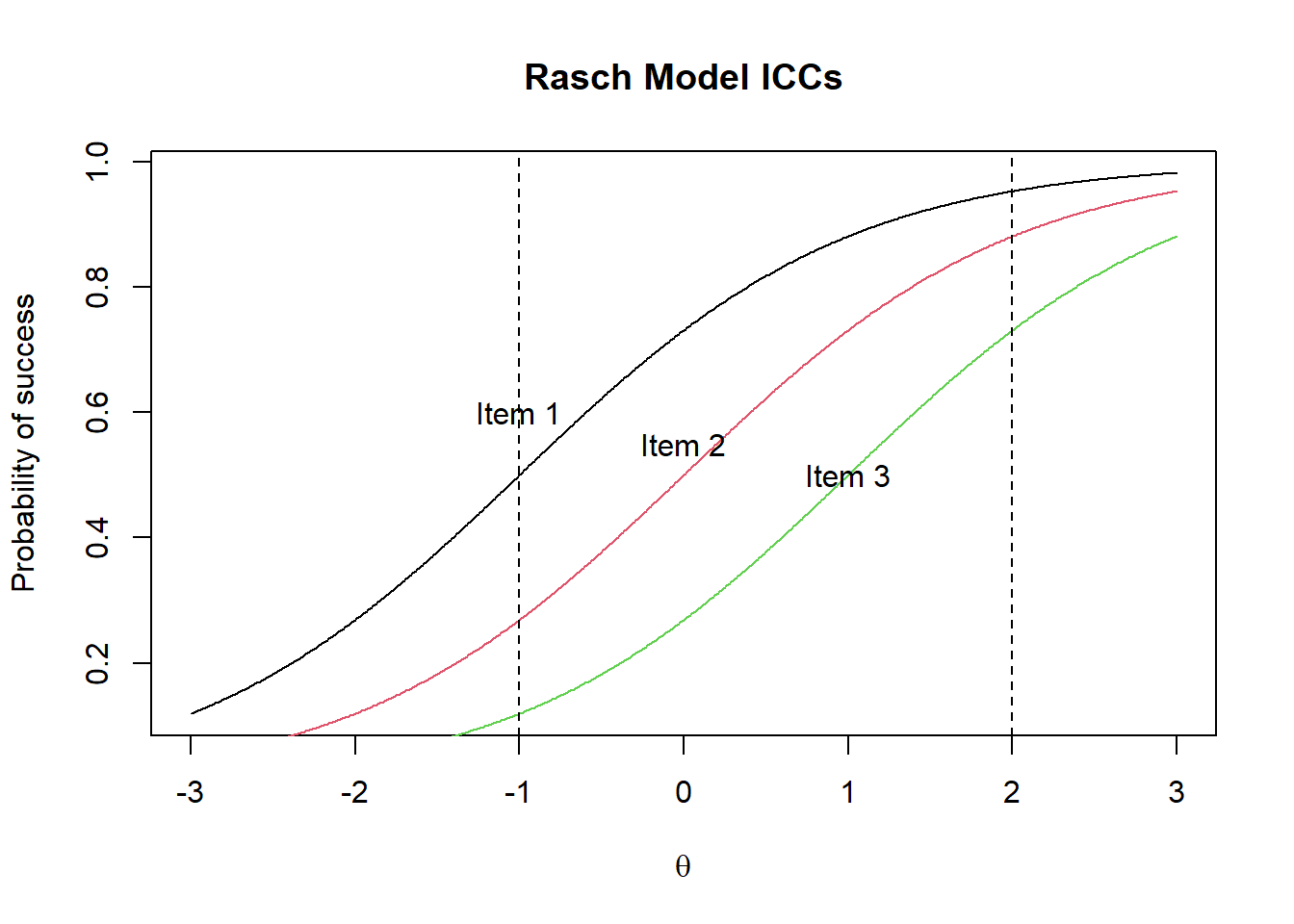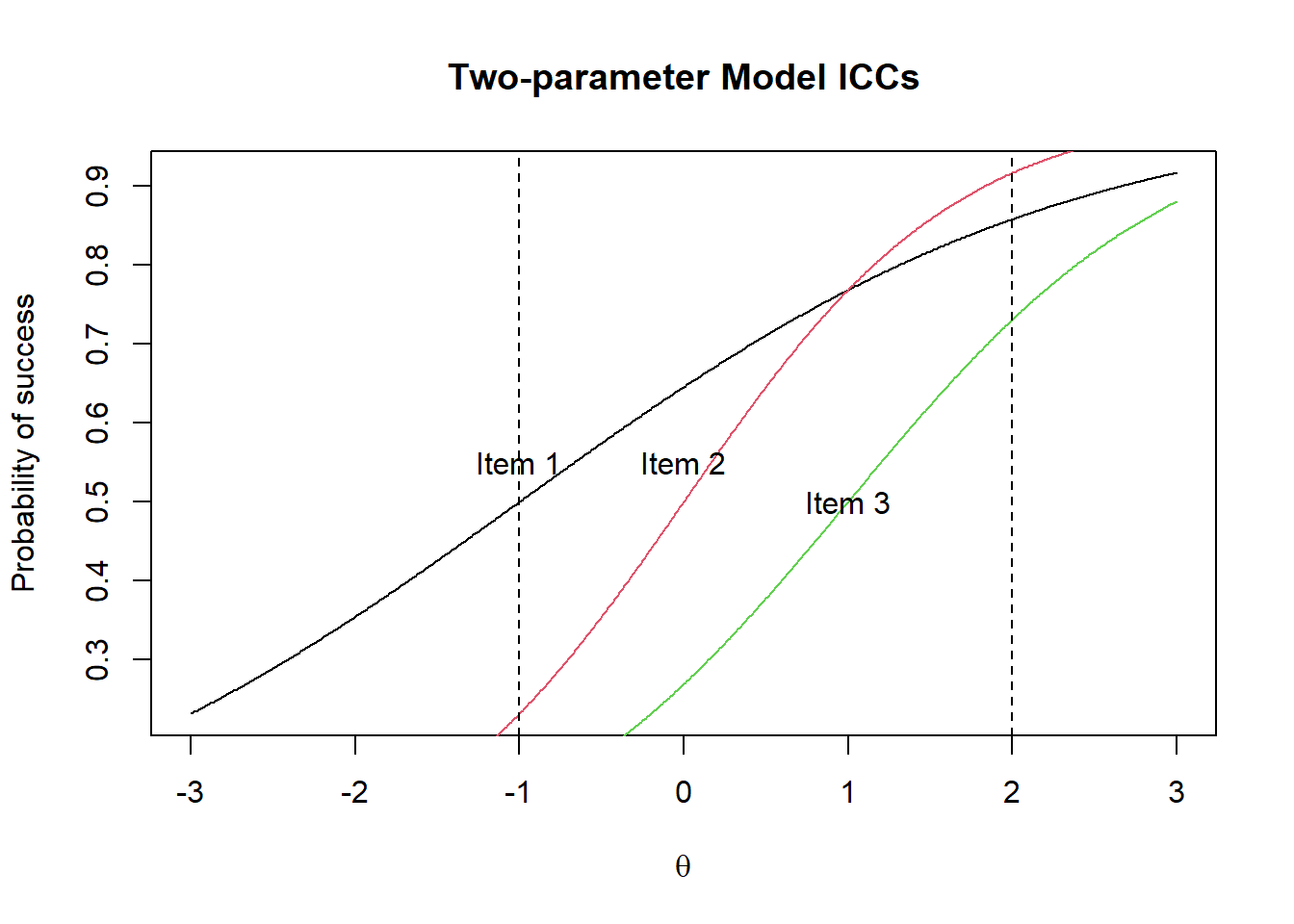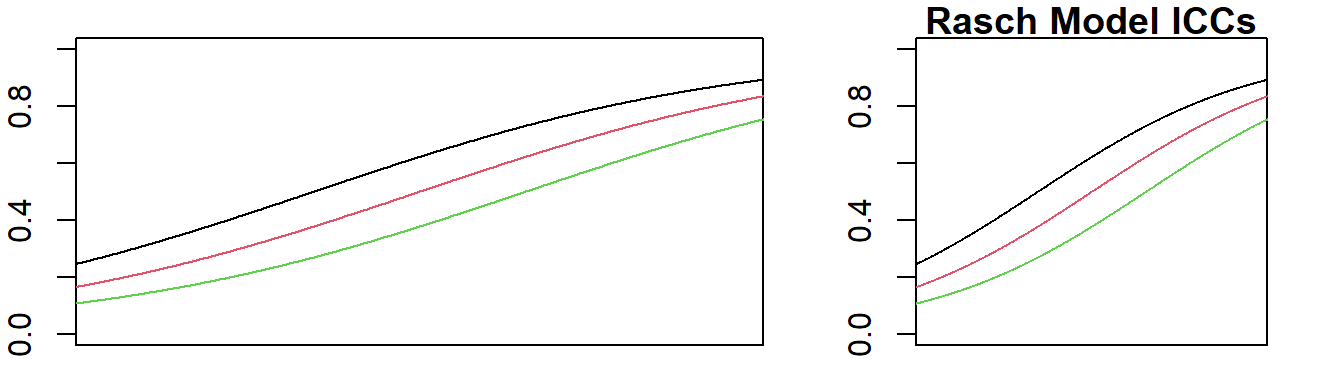# Chapter 3 Introduction to IRT

Under the IRT framework, a mathematical function is used to model the probability of success of a person on an item, given the person’s ability measure $$\theta$$ and the item’s difficulty measure $$\delta$$. This function should be bounded by 0 and 1, as it models probability. The function should be increasing as ability increases, indicating that the chance of success is higher for higher ability persons. When this probability is plotted as a function of $$\theta$$ (ability), the function should be S-shaped, with the left hand tail of the curve close to zero, rising to values close to 1 but under 1 on the right-side of the curve. Many mathematical functions fit this shape. In particular, cumulative distribution functions (cdf) or ogives have this S-shape and are bounded between 0 and 1. As an example, the logistic ogive and the normal ogive have both been used to model this probability. In the following, we will focus on the logistic ogive, or logistic cdf, to model this probability.

## 3.1 The Rasch Model

The Rasch item response model uses the following function to model the probability of success on an item with item difficulty $$\delta$$ and ability $$\theta$$:

$\begin{equation} Prob(X=1) = \frac{\exp(\theta - \delta)}{1+\exp(\theta - \delta)}\tag{3.1} \end{equation}$

where $$X$$ is the person’s score on the item, taking values of 0 or 1.

As an exercise for writing R code and for using the plot function in R, we will calculate and plot the probability function in (3.1).

rm(list=ls())  #remove all objects in the R environment

# Calculate Rasch model probability
delta <- 0.6  #item difficulty is 0.6
theta <- 1.0  #person ability is 1.0
prob <- exp(theta-delta)/(1+exp(theta-delta))
prob

Change the values of delta and theta, and observe how prob changes. In particular, try values when (1) delta = theta, (2) delta > theta, and (3) delta < theta. What happens when theta is very high, and when theta is very low?

We will plot the probability in (3.1) as a function of $$\theta$$.

#Calculate prob as a function the theta
delta <- 1 #item difficulty is 1
theta <- seq(-3,3,0.01) #a vector of theta from -3 to 3 in steps of 0.01
prob <- exp(theta-delta)/(1+exp(theta-delta)) #Calculate prob as a function of theta
plot(theta,prob,type="l") #Note the type is the letter l, not numeral 1.Figure 3.1: Item Characteristic Curve

## 3.2 Exercise

As an exercise, plot three ICC for three items with (1) $$\delta=-1$$, (2) $$\delta=0$$, and (3) $$\delta=1$$. Use three variables prob1, prob2 and prob3 to store the probabilities for the three items. The three graphs can be plotted in one picture by using the plot command for the first graph, and lines command for the second and third graph. For example,

plot(theta, prob1, type="l")
lines(theta, prob2)
lines(theta, prob3)

See if you can work out how to plot the three graphs using different colors. Is the ICC of the easier item on the left side or right side of the picture?

## 3.3 Theta Scale Unit

The $$\theta$$ scale is in “logit” unit. Answer the following questions, assuming the item responses follow a Rasch model:

1. What is the probability of success when $$\theta=\delta$$?
2. What is the probability of success when $$\theta=2$$ logit and $$\delta=1$$ logit?
3. What is the probability of success when a person’s ability is 1.5 logit higher than the item difficulty?
4. What is the probability of success when a person’s ability is 0.6 logit lower than the item difficulty?
5. Is the following statement TRUE or FALSE: The difference in logit between a person’s ability and an item’s difficulty determines the probability of success, irrespective of what the ability is.
6. Consider CTT item difficulty and person ability, can we say anything about the likelihood of success for a person who scored 80% on a test, on an item where 80% of the candidates answered correctly?

## 3.4 Parallel ICCs

Under the Rasch model, the ICCs of items are “parallel.” They have exactly the same shape, but they are placed at different points along the ability scale. These ICCs never cross each other. Consequently the order of item difficulties is the same for all persons. If Item 2 is more difficult than Item 1, then everyone finds Item 2 more difficult than Item 1 irrespective of the persons’ abilities. This is an important property of the Rasch model.

Figure 3.2 shows the Rasch model ICCs which are parallel, while Figure 3.3 shows the two-parameter model where the ICCs are not parallel.Figure 3.2: Rasch Model ICCs

Under the Rasch model, for any person, Item 1 is easier than Item 2 which is easier than item 3.

In Figure 3.3, the two-parameter ICCs are plotted, showing that the ICCs are not parallel.Figure 3.3: Two-parameter Model ICCs

Figure 3.3 shows that for a person with ability measure of -1 logit, Item 1 is the easiest item. But for a person with an ability of 2 logit, Item 2 is the easiest item.

## 3.5 Two-parameter IRT model

The graph in Figure 3.3 shows that the ICCs have different slopes (or steepness), with Item 2 having the steepest curve. Item 2 is the most discriminating item among the three items, while Item 1 is the least discriminating. An item with a flat curve does not distinguish high and low ability students well, since both high and low ability students will have more similar chances of obtaining the correct answer. In contrast, if an item has a steep slope (high discrimination), high ability students will have much higher chances of obtaining the correct answer than low ability students.

Mathematically, the two-parameter IRT model has a discrimination parameter in addition to the item difficulty parameter:

$\begin{equation} Prob(X=1) = \frac{\exp(a(\theta - \delta))}{1+\exp(a(\theta - \delta))}\tag{3.2} \end{equation}$

where $$a$$ is called the discrimination parameter.

## 3.6 Relative slope (discrimination) for the Rasch model

In the Rasch model, all discrimination parameters, $$a$$, are set to be equal. However, the Rasch model does not stipulate a fixed value for the discrimination parameter, except that they should be the same. Typically, when the parameters are estimated, many software programs set the discrimination parameter, $$a$$, to 1. If the set of items are highly discriminating items, the ability ($$\theta$$) scale will be stretched, leading to a large variance of $$\theta$$ and high test reliability. On the other hand, if the set of items are not highly discriminating items, the variance of $$\theta$$ will be small and the test reliability will be low.

Figure 3.4 shows that for a set of high discriminating items, the ICCs plotted have the scale stretched, so visually the ICCs do not look like they are very steep.Figure 3.4: Items with high discrimination (left) have stretched scale (right) to look flatter

Figure 3.5 shows that for a set of low discriminating items, the ICCs plotted have the scale shrunken, so the ICCs look steeper.Figure 3.5: Items with low discrimination (left) have shrunken scale (right) to look steeper

Consequently, if the data fit the Rasch model (i.e., parallel ICCs), it is not sufficient for us to conclude that the test items are of high quality. We still need to check the magnitude of the variance and test reliability. A visual impression of the ICCs does not tell us whether the items do a good job in separating students.

Clearly, theoretically, the Rasch model (also known as the one-parameter model) has nice properties. However, in real-life, test items rarely have equal discrimination. So the choice of an IRT model is not such a clear-cut decision. The good thing is, for most purposes, there will not be huge differences whether we use the Rasch model or the two-parameter model.

## 3.7 Homework

Plot three ICCs on the same graph, showing three items with different discrimination parameters fitting the 2-PL item response model.

### References

Rasch, Georg. 1960. Probabilistic Models for Some Intelligence and Attainment Tests. Copenhagen, Denmark: Danish Institute for Educational Research.